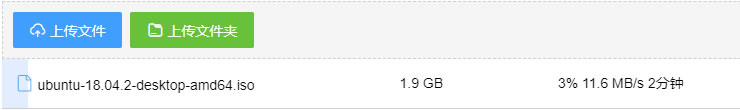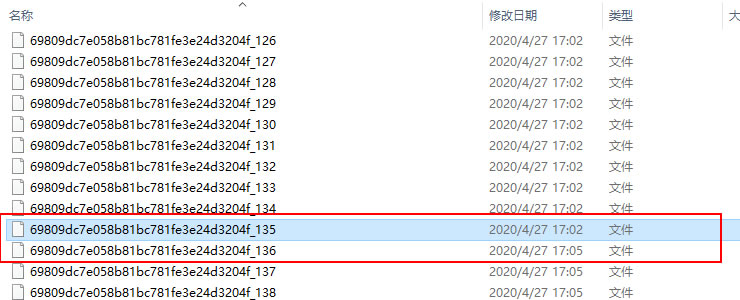## 文件上传之断点续传和跨端续传

#### 前端发送续传请求

``````// 服务器分片校验函数
let obj = JSON.parse(message);
if (obj.isExist) {
this.statusTextMap.success = '秒传文件';
return true;
}

return (obj.uploaded || []).indexOf(chunk.offset + 1) >= 0
},
``````

#### 后端分析断点，接受续传

``````//检测断点和md5
public function checkFile()
{
\$identifier = \$this->fileInfo['identifier'];
\$filePath = self::\$tmpDir. DIRECTORY_SEPARATOR . \$identifier; //临时分片文件路径
\$totalChunks = \$this->fileInfo['totalChunks'];

//检测文件md5是否已经存在
\$rs = \$this->checkMd5(\$identifier, \$this->fileInfo['totalSize']);
if (\$rs['isExist'] === true) {
return \$rs;
}

//检查分片是否存在
\$chunkExists = [];
for (\$index = 1; \$index <= \$totalChunks; \$index++ ) {
if (file_exists("{\$filePath}_{\$index}")) {
array_push(\$chunkExists, \$index);
}
}
if (count(\$chunkExists) == \$totalChunks) { //全部分片存在，则直接合成
\$this->merge();
} else {
return \$res;
}
}
``````

#### 试验#### 关于文件上传的总结

1条评论